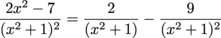## Partial Fractions

Have you ever looked at an algebraic fraction and wondered if there was a simpler way to put it? No? We didn't think so, but there are situations that you would need to simplify an algebraic fraction when you start studying calculus. In this section, we will teach you the nuts and bolts of how to simplify or decompose an algebraic fraction into simpler sums.

What you are doing here is prepping your brain for what's to come. Just like when you had to learn how to do addition and subtraction before you could solve an algebraic equation. Brain prep commence.

Let's go over the rules of decomposing an algebraic fraction. An algebraic fraction has an algebraic expression in the numerator and the denominator like this: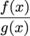Decomposing Partial Fractions: The Rules

1. Completely factor g(x) into factors if you can. Keep multiple factors in exponential forms.

2. If there are factors that looks like (mx + b)u, setup partial fractions like this: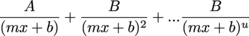3. If there are factors that look like (ax2 + bx + c)u, setup partial fractions like this: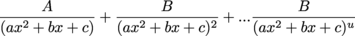4. Clear fractions by multiplying each side of the equation by the denominator of the left side.

5. Choose values of x that will cancel out terms to solve your constants A, B, C, etc. Substitute in and solve for the constants in you can. Repeat this step if necessary.

6. If you can't solve for a constant, choose another value of x and setup a system of equations.

7. If you have to setup a system of equations to solve for constants A, B, C, etc., try using inverse matrix operations to solve.

### Sample Problem

Setup the following fraction into terms with constants using the decomposition rules.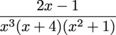Let's pick this apart piece by piece. Starting with the x3 factor on the bottom, we will need: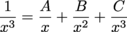The next factor on bottom that needs to be dealt with is the (x + 4).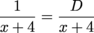Finally, the (x2 + 1) will be decomposed like this: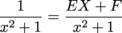The terms for the partial fraction decomposition will now look like this: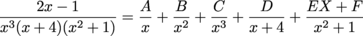Do you feel your brain cells multiplying? We don't know the value of the constants (A or B, etc) yet, but the next few sample problems will show you how to find the values of the constants.

### Sample Problem

Setup the terms for partial fractions, but don't solve for the constants.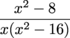Since we can factor the denominator a little bit, let's rewrite our fraction.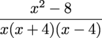And now (drum roll please), the equation for our partial fraction will look like this: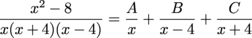### Sample Problem

Let's go back to our previous example and run through the steps to solving for the constants to get our actual partial fractions. We ended on this step:Going back to our instructions, we need to clear the fractions by multiplying each side by x(x + 4)(x – 4). That will make things look a bit simpler. (And at this point, simpler is good. We'll take what we can get.)

x2 – 8 = A(x – 4)(x + 4) + B(x)(x + 4) + C(x)(x – 4)

Our goal here is to find out what A, B, and C is to write our official partial fraction of this problem. We like the method of trying to cancel out terms. If x = 4, notice that the A and the C term would be 0, so let's try that first.Try x = -4:Try x = 0:Finally, we can write our partial fraction composition because we now know the value of the constants.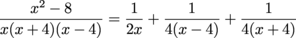We know that wasn't a simple process, but look at the right side of that equation. It looks much easier than the left side. In Calculus, you will be finding areas of functions (integration), and the three fractions on the right are much easier to integrate than the complicated algebraic fraction on the left.

### Sample Problem

Use partial fractions to simplify this fraction: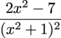First, we will write our terms with constants.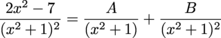Clearing the fraction on the left by multiplying each side by (x2 + 1)2, we get:

2x2 – 7 = A(x2 + 1) + B

Try x = 0:

2(0)2 – 7 = A((0)2 + 1) + B
-7 = A + B

Now don't get ahead of yourself. We didn't get to solve for A or B yet. We need to try another x value and setup a system of equations to find A and B.

Try x = 1:

2(1)2 – 7 = A((1)2 + 1) + B
-5 = 2A + B

So our system of equations is:

2A + B = -5
A + B = -7

We can set this up in a matrix problem to solve for A and B (remember, we did this in our earlier sections of this guide).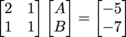Solving this, using inverse matrices, we get: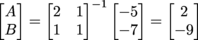Now, we are finally ready to state our partial fractions. (Phew!)